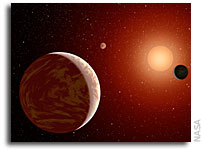# Analytical Models of Exoplanetary Atmospheres: Gaseous C-H-O-N Chemistry with 9 MoleculesWe present novel, analytical, equilibrium-chemistry formulae for the abundances of molecules in hot exoplanetary atmospheres that include the carbon, oxygen and nitrogen networks.

Our hydrogen-dominated solutions involve acetylene (C2H2), ammonia (NH3), carbon dioxide (CO2), carbon monoxide (CO), ethylene (C2H4), hydrogen cyanide (HCN), methane (CH4), molecular nitrogen (N2) and water (H2O). By considering only the gaseous phase, we prove that the mixing ratio of carbon monoxide is governed by a decic equation (polynomial equation of degree 10). We validate our solutions against numerical calculations of equilibrium chemistry that perform Gibbs free energy minimization and demonstrate that they are accurate for temperatures from 500--3000 K.

In hydrogen-dominated atmospheres, the ratio of abundances of HCN to CH4 is nearly constant across a wide range of carbon-to-oxygen ratios, which makes it a robust diagnostic of the metallicity in the gas phase. Our validated formulae allow for the convenient benchmarking of chemical kinetics codes and provide an efficient way of enforcing chemical equilibrium in atmospheric retrieval calculations.

Kevin Heng, Shang-Min Tsai
(Submitted on 17 Mar 2016)